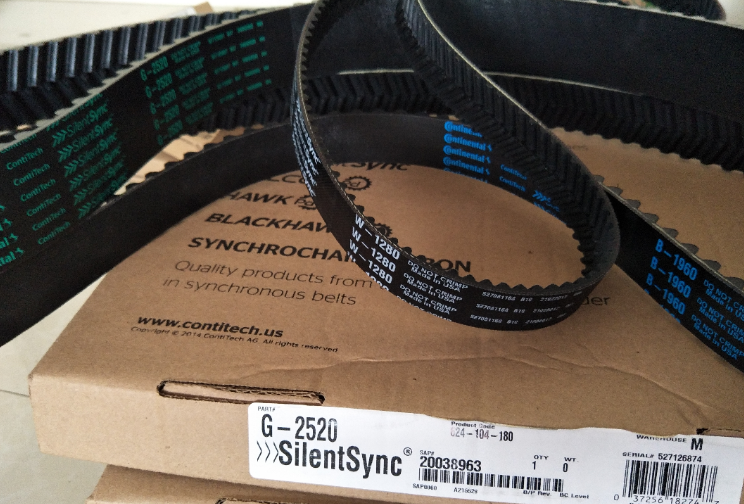# 人字齿同步带齿面载荷分布计算

2023-06-11 16:42 马牌

 Fk cos b × S b + Fk cos b × l = f R (cosa n - m sin an )(Qk - Qk +1 ) (2-24) AE AE 当带齿啮合干涉量sk   < 0 时， Fk cos b × S b + Fk -1 cos b × l = f R (cosa n - m sin an )(Qk   - Qk +1 ) (2-25) AE AE 将式(2-24)、(2-25)与带齿受力沿带齿节圆切线方向上的力平衡式联立，消去Qk 和Qk +1 ，得到如下带拉力的式子： 当带齿啮合干涉量sk   > 0 时， f cosq × F -1 - (2 f cosq + Sb cos b + l cos b ) × F + f cosq × F = 0 (2-26) R k R AE AE k R k +1 式(2-13)可用一个含定值系数的齐次线性二阶差分方程式表示： aFk -1 - bFk   + cFk +1   = 0 (2-27) 其中   a = f cosq ， b = 2 f cosq + Sb cos b + l cos b ，c = f cosq R R AE AE R 假定方程(2-27)具有F = r k ( r ¹ 0) 的解，再将它代入式(2-27)中，再用 rk +1 除等 k 式两边，得到下面的特征方程： a - b r + cr2 = 0 (2-28) 当b 2 - 4ac > 0 时，此特征方程有以下的特征根： 1 1 r = (b +   b 2 - 4ac ) ； r = (b -   b 2 - 4ac ) 1 2c 2 2c

 F = c r k + c rk (2-29) k 1   1 2 2 只要代入啮合的第1 号带齿和第n 号带齿的初始条件，就可以由下式来确定任 意常数c 1 和c 2 。将c 1 和c 2 代入式(2-29)后得到式(2-27)的解。 Fk = X k F0 +Yk Fn (2-30) 其中   X = C r k - Drk ，Y   = Ar k   - Brk ，   A = br -cr2 ，   B = br -cr2   ， 2 1 2 1 k BC - AD k BC - AD 11 22 C = a r n -1 -brn ， D = a r n -1 -brn 1 1 2 2 。

k 个带齿上的载荷可通过力平衡关系式得到，表达如下：

 Q   = Fk -1 cos q - Fk cosq (2-31) k cos a n - m sinan 带齿顶部的正压力表示为： N k   = Fk -1 sinq + Fk sinq - Qk sin a n - m Qk cosan (2-32) 当带齿啮合干涉量sk   < 0 时， ( f cosq + l cos b ) × F - (2 f cosq - Sb cos b ) × F + f cosq × F = 0 (2-33) R R R AE k -1 AE k k +1 式(2-33)亦可用一个定值系数的齐次线性二阶差分方程式表示： a ' F - b' F + c ' F = 0 (2-34) k -1 k k +1 其中   a '   = fR cosq + l cos b ，b ' = 2 fR cosq - Sb cos b ，c ' = fR cosq AE AE 解方程式(2-34)同样可得第k 号带齿拉力Fk 的表达式，继而可根据带齿受力的 平衡关系式求出第k 号带齿载荷的载荷表达式如下： Q   = Fk cos q - Fk -1 cosq (2-35) k cos a n - m sinan 带齿顶部的正压力表示为： N k   = Fk -1 sinq + Fk sinq - Qk sin a n - m Qk cosan (2-36)

 Q   = F0 cos q - F1 cosq = (1 - X 1 )F0 cos q -Y1 Fn cosq (2-37) max cos a n - m sin a n cos a n - m sinanSYNCHROFLEX同步带 传动带 德国马牌同步带 Synchroflex皮带 同步带轮 KUKA同步带中国总代理官网